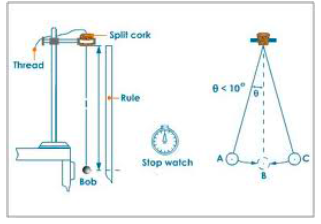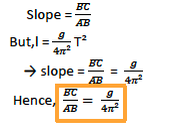Home PHYSICS TOPIC 6: MOTION IN STRAIGHT LINE | PHYSICS FORM 1

# TOPIC 6: MOTION IN STRAIGHT LINE | PHYSICS FORM 1

230
0
SHARE### TOPIC 6: MOTION IN STRAIGHT LINE | PHYSICS FORM 1

Motion In Straight Line

Motion is the change of position of an object from one place to another. There are two types of motions; i.Circular motion-Is the motion of an object in a circle. Examples; a/. motion of the electron around the nucleus of an atom b/.revolutionary movement of the earth around the sun. ii.Linear motion-Is the motion of an object in a straight line.
Difference between Distance and Displacement
Distinguish between Distance and Displacement
Distance
Is the length between two points or two objects.It is a scalar quantity
i.e. it has a magnitude only but no direction. Its symbol is X or S and
the SI unit is meter (m). Other units used are Kilometer (km) and
Centimeter (cm)
Displacement Is the distance in a specific direction. It is a vector quantity i.e. it has both magnitude and direction.
Differences between distance and displacement
 Distance Displacement Is the length moved by an object between two points Is the distance in a specific direction It is a scalar quantity It is a vector quantity
The SI Units of Distance and Displacement
State the SI units of Distance and Displacement
The symbol for distance is X or S and the SI unit is meter (m). Other units used are Kilometer (km) and Centimeter (cm)
The standard unit of displacement in the International System of Units ( SI ) is the meter (m).
The Concept of Gravitational Force
Explain the concept of gravitational force
The
acceleration of a free falling body is known as the acceleration due to
gravity denoted by g and controlled gy gravitational force of the
earth.
When
two bodies of different masses are released from a certain height h
above the ground, they will reach the ground at different times with the
heavier one reaching earlier before the lighter one.
The
reason is that the air resistance is more effective on lighter bodies
than in heavier bodies, consequently this affect acceleration due to
gravity in a reverse manner.
But
dropping a light object and a heavy object in a vacuum they will reach
the ground at the same time due to the absence of air resistance effect.
Consider the following two cases;
Case I
Consider a body falling freely from a certain height h and uses time t to reach the ground.In this case: acceleration, a = acceleration due to gravity g
Initial velocity, u = 0
Final velocity, v.Case II
Consider
a body thrown vertically upwards from the ground with an initial
velocity u to a certain height h and then comes back to the ground after
time t.In this case;
• Acceleration, a = -g
• Initial velocity = u
• Velocity at h = 0
• Final velocity at the ground = vAcceleration due to Gravity
Determine acceleration due to gravity
A
simple pendulum is a small heavy body suspended by a light inextensible
string from a fixed support and it is normally used to determine
acceleration due to gravity.
It
is made by attaching a a long thread to a spherical ball called a
pendulum bob. The string is held at a fixed at a fixed support like two
pieces of wood held by a clamp and stand.
If
the bob is slightly displaced to position B,it swings to and from going
to C through O and back to B through O. When the pendulum complete one
cycle/revolution the time taken is called the period of oscillation, T.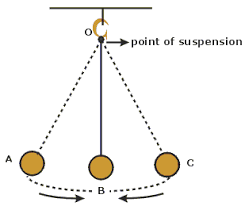Definitions
1. Period ,T is the time taken by the pendulum bob to complete one complete cycle.
2. Angular displacement is the angle made between the string and the vertical axis when the bob is displaced to a maximum displacement.
3. Amplitude is the maximum displacement by which the pendulum has been displaced.
4. Length of pendulum is the length of the string from the point of attachment on the wooden pieces to the canterof gravity of the bob.
From
the experiments, it has been observed that, changing the weight of the
bob and keeping the same length of pendulum, the period is always
constant provided that all swings are small though they may be different
in size.
The period T of the pendulum is given by;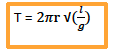Where; l = length of pendulum; g = acceleration due to gravity
Also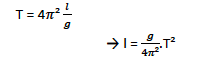It follows that if we plot a graph of l against T. it is going to be a straight line with a slope g/4Π2and y –intercept equal to 0.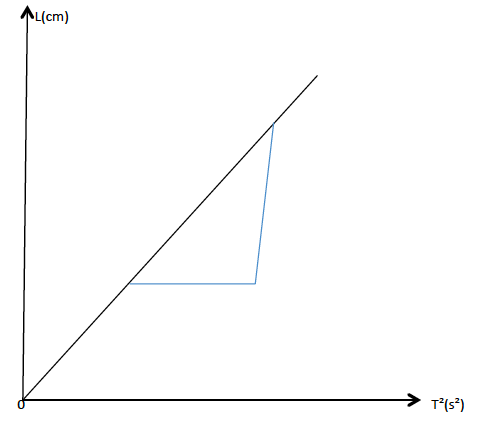When
the bob is raised to point B it will gain potential energy and the bob
will swing due to the conservation of energy from potential to kinetic
energy.
• At B and C all energy is P.E.
• At O all energy is K.E.
• P.E at B = K.E at O.
If
the pendulum swung in vacuum the oscillations would have been
continuous. But practically, air friction causes losses of energy of the
pendulum bob. That is why after a certain time the oscillations cease.
The Application of Gravitational Force
Explain the application of gravitational force
Activity 2
Experiment
Aim: Determination of acceleration due to gravity by using the simple pendulum.
Materials and apparatus: A simple pendulum, stand, clamps and stop watch.
Procedures